## NAME

r.futures.pga - Simulates landuse change using FUTure Urban-Regional Environment Simulation (FUTURES).
Module uses Patch-Growing Algorithm (PGA) to simulate urban-rural landscape structure development.

## KEYWORDS

raster, patch growing, urban, landscape, modeling

## SYNOPSIS

r.futures.pga
r.futures.pga --help
r.futures.pga [-s] developed=name subregions=name [subregions_potential=name] predictors=name[,name,...] development_pressure=name n_dev_neighbourhood=integer development_pressure_approach=string gamma=float scaling_factor=float output=name [output_series=basename] devpot_params=name demand=name [separator=character] patch_sizes=name num_neighbors=integer discount_factor=float seed_search=string compactness_mean=float compactness_range=float [num_steps=integer] [potential_weight=name] [incentive_power=float] [random_seed=integer] [memory=float] [--overwrite] [--help] [--verbose] [--quiet] [--ui]

### Flags:

-s
Generate random seed (result is non-deterministic)
Automatically generates random seed for random number generator (use when you don't want to provide the seed option)
--overwrite
Allow output files to overwrite existing files
--help
Print usage summary
--verbose
Verbose module output
--quiet
Quiet module output
--ui
Force launching GUI dialog

### Parameters:

developed=name [required]
Raster map of developed areas (=1), undeveloped (=0) and excluded (no data)
subregions=name [required]
Raster map of subregions
subregions_potential=name
Raster map of subregions used with potential file
If not specified, the raster specified in subregions parameter is used
predictors=name[,name,...] [required]
Names of predictor variable raster maps
Listed in the same order as in the development potential table
development_pressure=name [required]
Raster map of development pressure
n_dev_neighbourhood=integer [required]
Size of square used to recalculate development pressure
development_pressure_approach=string [required]
Approaches to derive development pressure
Options: occurrence, gravity, kernel
Default: gravity
gamma=float [required]
Influence of distance between neighboring cells
scaling_factor=float [required]
Scaling factor of development pressure
output=name [required]
State of the development at the end of simulation
output_series=basename
Basename for raster maps of development generated after each step
Name for output basename raster map(s)
devpot_params=name [required]
CSV file with development potential parameters for each region
Each line should contain region ID followed by parameters (intercepts, development pressure, other predictors). First line is ignored, so it can be used for header
demand=name [required]
CSV file with number of cells to convert for each step and subregion
separator=character
Field separator
Separator used in input CSV files
Default: comma
patch_sizes=name [required]
File containing list of patch sizes to use
num_neighbors=integer [required]
The number of neighbors to be used for patch generation (4 or 8)
Options: 4, 8
Default: 4
discount_factor=float [required]
Discount factor of patch size
seed_search=string [required]
The way location of a seed is determined (1: uniform distribution 2: development probability)
Options: random, probability
Default: probability
compactness_mean=float [required]
Mean value of patch compactness to control patch shapes
compactness_range=float [required]
Range of patch compactness to control patch shapes
num_steps=integer
Number of steps to be simulated
potential_weight=name
Raster map of weights altering development potential
Values need to be between -1 and 1, where negative locally reducesprobability and positive increases probability.
incentive_power=float
Exponent to transform probability values p to p^x to simulate infill vs. sprawl
Values > 1 encourage infill, < 1 urban sprawl
Options: 0-10
Default: 1
random_seed=integer
Seed for random number generator
The same seed can be used to obtain same results or random seed can be generated by other means.
memory=float
Memory in GB

## DESCRIPTION

Module r.futures.pga is part of FUTURES land change model. This module uses stochastic Patch-Growing Algorithm (PGA) and a combination of field-based and object-based representations to simulate land changes. PGA simulates undeveloped to developed land change by iterative site selection and a contextually aware region growing mechanism. Simulations of change at each time step feed development pressure back to the POTENTIAL submodel, influencing site suitability for the next step.

### Patch growing

Patches are constructed in three steps. First, a potential seed is randomly selected from available cells. In case seed_search is probability, the probability value (based on POTENTIAL) of the seed is tested using Monte Carlo approach, and if it doesn't survive, new potential seed is selected and tested. Second, using a 4- or 8-neighbor (see num_neighbors) search rule PGA grows the patch. PGA decides on the suitability of contiguous cells based on their underlying development potential and distance to the seed adjusted by compactness parameter given in compactness_mean and compactness_range. The size of the patch is determined by randomly selecting a patch size from patch sizes file and multiplied by discount_factor. To find optimal values for patch sizes and compactness, use module r.futures.calib. Once a cell is converted, it remains developed. PGA continues to grow patches until the per capita land demand is satisfied.

### Development pressure

Development pressure is a dynamic spatial variable derived from the patch-building process of PGA and associated with the POTENTIAL submodel. At each time step, PGA updates the POTENTIAL probability surface based on land change, and the new development pressure then affects future land change. The initial development pressure is computed using module r.futures.devpressure. The same input parameters of this module (gamma, scaling factor and n_dev_neighbourhood) are then used as input for r.futures.pga.

### Scenarios

Scenarios involving policies that encourage infill versus sprawl can be explored using the incentive_power parameter, which uses a power function to transform the probability in POTENTIAL.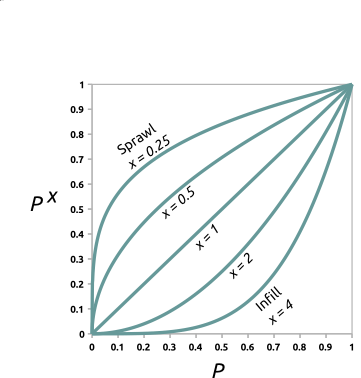Figure: Transforming development potential surface using incentive tables with different power functions.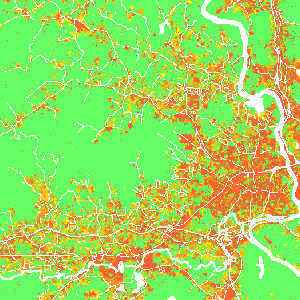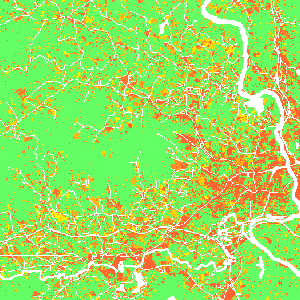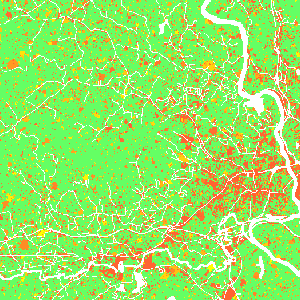Figure: Effect of incentive table on development probability: infill (left), status quo (middle), sprawl (right) scenario.

Additionally, parameter potential_weight (raster map from -1 to 1) enables to include policies (such as new regulations or fees) which limit or encourage development in certain areas. Where potential_weight values are lower than 0, the probability surface is simply multiplied by the values, which results in decreased site suitability. Similarly, values greater than 0 result in increased site suitability. The probability surface is transformed from initial probability p with value w to p + w - p * w.

### Output

After the simulation ends, raster specified in parameter output is written. If optional parameter output_series is specified, additional output is a series of raster maps for each step. Cells with value 0 represents the initial development, values >= 1 then represent the step in which the cell was developed. Undeveloped cells have value -1.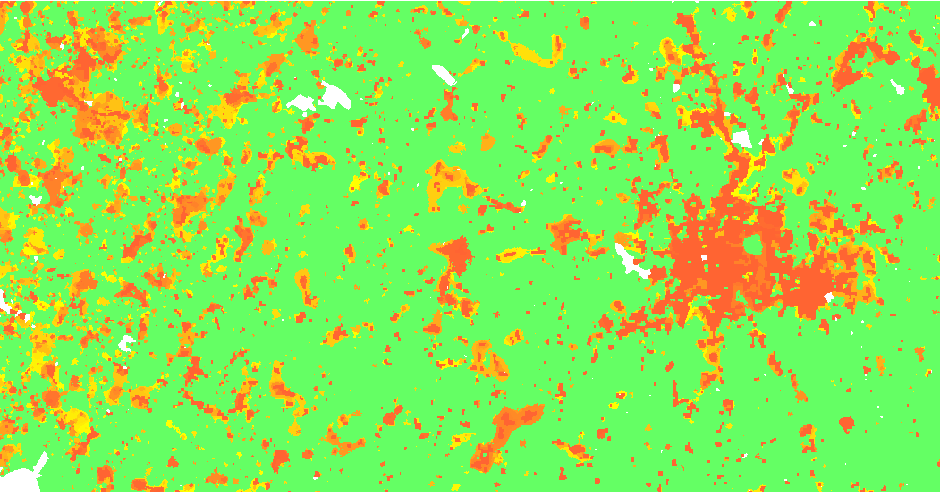Figure: Output map of developed areas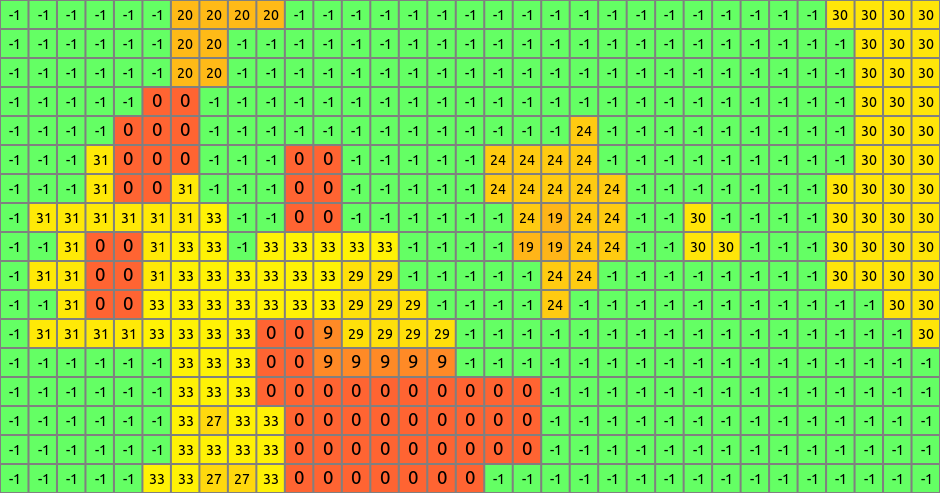Figure: Detail of output map

## EXAMPLE

```r.futures.pga -s developed=lc96 predictors=d2urbkm,d2intkm,d2rdskm,slope \
demand=demand.txt devpot_params=devpotParams.csv discount_factor=0.6 \
compactness_mean=0.4 compactness_range=0.08 num_neighbors=4 seed_search=probability \
patch_sizes=patch_sizes.txt development_pressure=gdp n_dev_neighbourhood=10 \
development_pressure_approach=gravity gamma=2 scaling_factor=1 \
subregions=subregions incentive_power=2 \
potential_weight=weight_1 output=final_results output_series=development
```

## REFERENCES

FUTURES, r.futures.devpressure, r.futures.calib, r.futures.demand, r.futures.potential, r.futures.parallelpga, r.sample.category

## AUTHORS

Corresponding author:
Ross K. Meentemeyer, rkmeente ncsu edu, Center for Geospatial Analytics, NCSU

Original standalone version:
Ross K. Meentemeyer *
Wenwu Tang *
Monica A. Dorning *
John B. Vogler *
Nik J. Cunniffe *
Douglas A. Shoemaker *
Jennifer A. Koch **

* Department of Geography and Earth Sciences, UNC Charlotte
** Center for Geospatial Analytics, NCSU

Port to GRASS GIS and GRASS-specific additions:
Anna Petrasova, NCSU GeoForAll
Vaclav Petras, NCSU GeoForAll

## SOURCE CODE

Available at: r.futures.pga source code (history)

Latest change: Thursday Jun 23 08:55:52 2022 in commit: de1f13a3f5382fcd17de2cbc8c1fa8dfaa87d21a

© 2003-2022 GRASS Development Team, GRASS GIS 8.2.0 Reference Manual